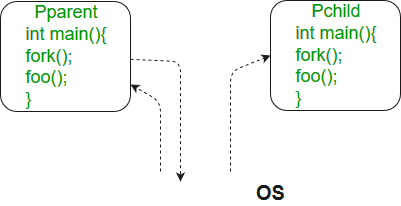# fork() in C

• Difficulty Level : Medium
• Last Updated : 09 Dec, 2019

Fork system call is used for creating a new process, which is called child process, which runs concurrently with the process that makes the fork() call (parent process). After a new child process is created, both processes will execute the next instruction following the fork() system call. A child process uses the same pc(program counter), same CPU registers, same open files which use in the parent process.

It takes no parameters and returns an integer value. Below are different values returned by fork().

Negative Value: creation of a child process was unsuccessful.
Zero: Returned to the newly created child process.
Positive value: Returned to parent or caller. The value contains process ID of newly created child process.Please note that the above programs don’t compile in Windows environment.

1. Predict the Output of the following program:.

 `#include ` `#include ` `#include ` `int` `main() ` `{ ` ` `  `    ``// make two process which run same ` `    ``// program after this instruction ` `    ``fork(); ` ` `  `    ``printf``(``"Hello world!\n"``); ` `    ``return` `0; ` `} `

Output:

```Hello world!
Hello world!
```
2. Calculate number of times hello is printed:

 `#include ` `#include ` `int` `main() ` `{ ` `    ``fork(); ` `    ``fork(); ` `    ``fork(); ` `    ``printf``(``"hello\n"``); ` `    ``return` `0; ` `} `

Output:

```hello
hello
hello
hello
hello
hello
hello
hello
```

The number of times ‘hello’ is printed is equal to number of process created. Total Number of Processes = 2n, where n is number of fork system calls. So here n = 3, 23 = 8

Let us put some label names for the three lines:

```fork ();   // Line 1
fork ();   // Line 2
fork ();   // Line 3

L1       // There will be 1 child process
/     \     // created by line 1.
L2      L2    // There will be 2 child processes
/  \    /  \   //  created by line 2
L3  L3  L3  L3  // There will be 4 child processes
// created by line 3

```

So there are total eight processes (new child processes and one original process).

If we want to represent the relationship between the processes as a tree hierarchy it would be the following:

The main process: P0
Processes created by the 1st fork: P1
Processes created by the 2nd fork: P2, P3
Processes created by the 3rd fork: P4, P5, P6, P7

```             P0
/   |   \
P1    P4   P2
/  \          \
P3    P6         P5
/
P7
```
3. Predict the Output of the following program:

 `#include ` `#include ` `#include ` `void` `forkexample() ` `{ ` `    ``// child process because return value zero ` `    ``if` `(fork() == 0) ` `        ``printf``(``"Hello from Child!\n"``); ` ` `  `    ``// parent process because return value non-zero. ` `    ``else` `        ``printf``(``"Hello from Parent!\n"``); ` `} ` `int` `main() ` `{ ` `    ``forkexample(); ` `    ``return` `0; ` `} `

Output:

```1.
Hello from Child!
Hello from Parent!
(or)
2.
Hello from Parent!
Hello from Child!
```

In the above code, a child process is created. fork() returns 0 in the child process and positive integer in the parent process.
Here, two outputs are possible because the parent process and child process are running concurrently. So we don’t know whether the OS will first give control to the parent process or the child process.

Important: Parent process and child process are running the same program, but it does not mean they are identical. OS allocate different data and states for these two processes, and the control flow of these processes can be different. See next example:

4. Predict the Output of the following program:

 `#include ` `#include ` `#include ` ` `  `void` `forkexample() ` `{ ` `    ``int` `x = 1; ` ` `  `    ``if` `(fork() == 0) ` `        ``printf``(``"Child has x = %d\n"``, ++x); ` `    ``else` `        ``printf``(``"Parent has x = %d\n"``, --x); ` `} ` `int` `main() ` `{ ` `    ``forkexample(); ` `    ``return` `0; ` `} `

Output:

```Parent has x = 0
Child has x = 2
(or)
Child has x = 2
Parent has x = 0
```

Here, global variable change in one process does not affected two other processes because data/state of two processes are different. And also parent and child run simultaneously so two outputs are possible.

fork() vs exec()

The fork system call creates a new process. The new process created by fork() is a copy of the current process except for the returned value. The exec() system call replaces the current process with a new program.

Exercise:

1. A process executes the following code:

 `for` `(i = 0; i < n; i++) ` `    ``fork(); `

The total number of child processes created is: (GATE-CS-2008)
(A) n
(B) 2^n – 1
(C) 2^n
(D) 2^(n+1) – 1;

See this for solution.

2. Consider the following code fragment:

 `if` `(fork() == 0) { ` `    ``a = a + 5; ` `    ``printf``(``"%d, %d\n"``, a, &a); ` `} ` `else` `{ ` `    ``a = a –5; ` `    ``printf``(``"%d, %d\n"``, a, &a); ` `} `

Let u, v be the values printed by the parent process, and x, y be the values printed by the child process. Which one of the following is TRUE? (GATE-CS-2005)
(A) u = x + 10 and v = y
(B) u = x + 10 and v != y
(C) u + 10 = x and v = y
(D) u + 10 = x and v != y
See this for solution.

3. Predict output of below program.

 `#include ` `#include ` `int` `main() ` `{ ` `    ``fork(); ` `    ``fork() && fork() || fork(); ` `    ``fork(); ` ` `  `    ``printf``(``"forked\n"``); ` `    ``return` `0; ` `} `

See this for solution

This article is contributed by Team GeeksforGeeks and Kadam Patel. If you like GeeksforGeeks and would like to contribute, you can also write an article using contribute.geeksforgeeks.org or mail your article to contribute@geeksforgeeks.org. See your article appearing on the GeeksforGeeks main page and help other Geeks.